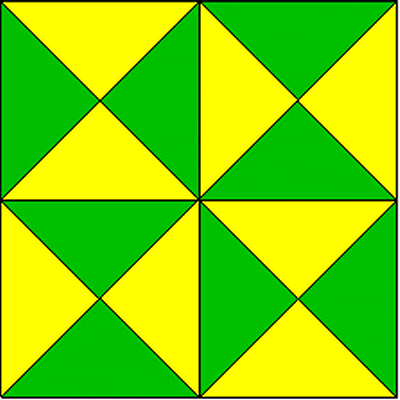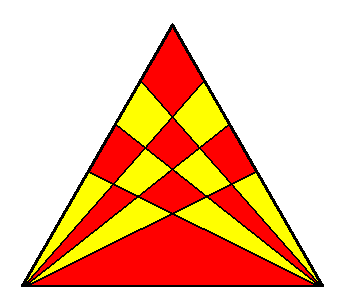May 20, 2019, 03:23:19 AM

## News:

Welcome to Xhanch Forum

## How many triangles are there?

Started by Xhanch Studio, April 17, 2011, 05:57:57 AM

Go Down

#### Xhanch Studio

#####April 17, 2011, 05:57:57 AMLast Edit: April 17, 2011, 06:35:30 AM by Xhanch Studio
Well, these puzzles are quite simple.
You just need to count how many triangles are there.
Note: don't peek the answers before trying to solve the puzzle on your ownHere they are:

• [spoiler=Type 1][spoiler=The answer]There are 27 triangles here. The breakdown:

• X1 triangles: 16

• X4 triangles: 7

• X9 triangles: 3

• X16 triangles: 1

Math solution: T(n) = floor(n * (n + 2) * (2n + 1) / 8)
n = 4
T(4) = floor(4 * (4 + 2) * (2*4 + 1) / 8) = 27[/spoiler][/spoiler]

• [spoiler=Type 2][spoiler=The answer]There are 48 triangles here.

Math solution: T(n) = floor(n * (n + 2) * (2n + 1) / 8)
n = 5
T(5) = floor(5 * (5 + 2) * (2*5 + 1) / 8) = 48[/spoiler][/spoiler]

• [spoiler=Type 3][spoiler=The answer]There are 44 triangles here. The breakdown:

• X1 triangles: 16

• X2 triangles: 16

• X4 triangles: 8

• X8 triangles: 4

[/spoiler][/spoiler]

• [spoiler=Type 4][spoiler=The answer]There are 64 triangles here. [spoiler=The breakdown:][/spoiler][/spoiler][/spoiler]

• [spoiler=Type 5][spoiler=The answer]There are 35 triangles here. The breakdown:

• X1 triangles: 10

• X2 triangles: 10

• X3 triangles: 10

• X5 triangles: 5

[/spoiler][/spoiler]

• [spoiler=Type 6][spoiler=The answer]There are 8292 triangles here.[/spoiler][/spoiler]

• [spoiler=Type 7][spoiler=The answer]There are 92 triangles here.[/spoiler][/spoiler]

Best Regards,
Susanto B.Sc
----------------------------------------------------------------------------
Web development services, WordPress plugin and theme development, PSD to XHTML conversion - http://xhanch.com
Read free manga online - http://authrone.com

Go Up